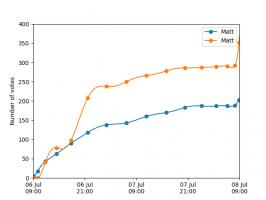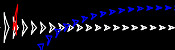mscroggs.co.uk
mscroggs.co.uksubscribe

# Blog

2018-09-13
This is a post I wrote for round 2 of The Aperiodical's Big Internet Math-Off 2018. As I went out in round 1 of the Big Math-Off, you got to read about the real projective plane instead of this.
Polynomials are very nice functions: they're easy to integrate and differentiate, it's quick to calculate their value at points, and they're generally friendly to deal with. Because of this, it can often be useful to find a polynomial that closely approximates a more complicated function.
Imagine a function defined for $$x$$ between -1 and 1. Pick $$n-1$$ points that lie on the function. There is a unique degree $$n$$ polynomial (a polynomial whose highest power of $$x$$ is $$x^n$$) that passes through these points. This polynomial is called an interpolating polynomial, and it sounds like it ought to be a pretty good approximation of the function.
So let's try taking points on a function at equally spaced values of $$x$$, and try to approximate the function:
$$f(x)=\frac1{1+25x^2}$$
Polynomial interpolations of $$\displaystyle f(x)=\frac1{1+25x^2}$$ using equally spaced points
I'm sure you'll agree that these approximations are pretty terrible, and they get worse as more points are added. The high error towards 1 and -1 is called Runge's phenomenon, and was discovered in 1901 by Carl David Tolmé Runge.
All hope of finding a good polynomial approximation is not lost, however: by choosing the points more carefully, it's possible to avoid Runge's phenomenon. Chebyshev points (named after Pafnuty Chebyshev) are defined by taking the $$x$$ co-ordinate of equally spaced points on a circle.
The following GIF shows interpolating polynomials of the same function as before using Chebyshev points.
Nice, we've found a polynomial that closely approximates the function... But I guess you're now wondering how well the Chebyshev interpolation will approximate other functions. To find out, let's try it out on the votes over time of my first round Big Internet Math-Off match.
The graphs below show the results of the match over time interpolated using 16 uniform points (left) and 16 Chebyshev points (right). You can see that the uniform interpolation is all over the place, but the Chebyshev interpolation is very close the the actual results.Scroggs vs Parker, 6-8 July 2018, approximated using uniform points (left) and Chebyshev points (right)
But maybe you still want to see how good Chebyshev interpolation is for a function of your choice... To help you find out, I've written @RungeBot, a Twitter bot that can compare interpolations with equispaced and Chebyshev points. Just tweet it a function, and it'll show you how bad Runge's phenomenon is for that function, and how much better Chebysheb points are.
A list of constants and functions that RungeBot understands can be found here.

### Similar postsA surprising fact about quadrilateralsInteresting tautologiesBig Internet Math-Off stickers 2019Mathsteroids

Comments in green were written by me. Comments in blue were not written by me.